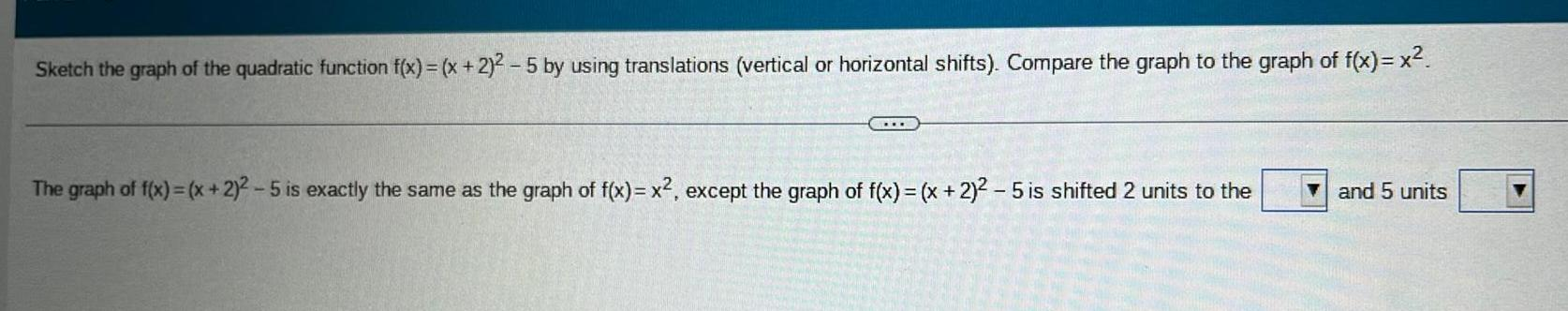Question:

# Sketch the graph of the quadratic function f x x 2 2 5 by

Last updated: 9/14/2023Sketch the graph of the quadratic function f x x 2 2 5 by using translations vertical or horizontal shifts Compare the graph to the graph of f x x E The graph of f x x 2 2 5 is exactly the same as the graph of f x x except the graph of f x x 2 2 5 is shifted 2 units to the and 5 units